https://doi.org/10.5194/angeo-24-941-2006
https://doi.org/10.5194/angeo-24-941-2006

# Comparison of large-scale Birkeland currents determined from Iridium and SuperDARN data

D. L. Green, C. L. Waters, B. J. Anderson, H. Korth, and R. J. Barnes

Abstract. The Birkeland currents, J||, electrically couple the high latitude ionosphere with the near Earth space environment. Approximating the spatial distribution of the Birkeland currents may be achieved using the divergence of the ionospheric electric field,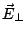, assuming zero conductance gradients such that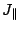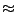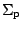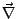. In this paper, electric field data derived from the Super Dual Auroral Radar Network (SuperDARN) are used to calculate, which is compared with the Birkeland current distribution derived globally from the constellation of Iridium satellites poleward of 60° magnetic latitude. We find that the assumption of zero conductance gradients is often a poor approximation. On the dayside, in regions where the SuperDARN electric field is constrained by radar returns, the agreement in the locations of regions of upward and downward current betweenand J|| obtained from Iridium data is reasonable with differences of less than 3° in the latitudinal location of major current features. It is also shown that away from noon, currents arising from conductance gradients can be larger than thecomponent. By combining theestimate in regions of radar coverage with in-situ estimates of conductance gradients from DMSP satellite particle data, the agreement with the Iridium derived J|| is considerably improved. However, using an empirical model of ionospheric conductance did not account for the conductance gradient current terms. In regions where radar data are sparse or non-existent and therefore constrained by the statistical potential model theapproximation does not agree with J|| calculated from Iridium data.

Share